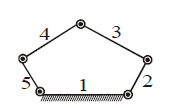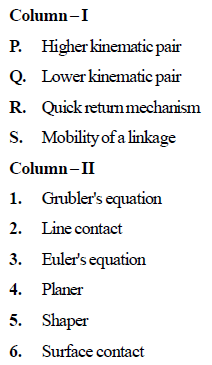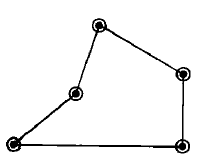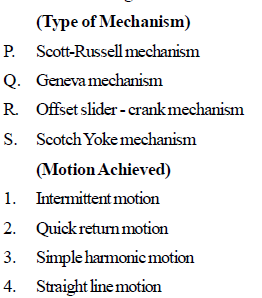Courses

# Test: Theory of Machines Level - 3

## 25 Questions MCQ Test Mechanical Engineering SSC JE (Technical) | Test: Theory of Machines Level - 3

Description
This mock test of Test: Theory of Machines Level - 3 for Mechanical Engineering helps you for every Mechanical Engineering entrance exam. This contains 25 Multiple Choice Questions for Mechanical Engineering Test: Theory of Machines Level - 3 (mcq) to study with solutions a complete question bank. The solved questions answers in this Test: Theory of Machines Level - 3 quiz give you a good mix of easy questions and tough questions. Mechanical Engineering students definitely take this Test: Theory of Machines Level - 3 exercise for a better result in the exam. You can find other Test: Theory of Machines Level - 3 extra questions, long questions & short questions for Mechanical Engineering on EduRev as well by searching above.
QUESTION: 1

Solution:
QUESTION: 2

Solution:
QUESTION: 3

### Calculate the thrust in connecting rod, if piston effort is 200 kN and crank makes an angle of 45ofrom TDC. Assume obliquity ratio = 3.5

Solution:
QUESTION: 4

Kinematic pairs are those which have two elements that

Solution:
QUESTION: 5

Which of the following is an inversion of singleslider- crank chain ?

Solution:
QUESTION: 6

Oldham's coupling is used to connect two shafts which are

Solution:
QUESTION: 7

A kinematic chain is known as mechanism when

Solution:
QUESTION: 8

Degree of freedom of super structure is

Solution:
QUESTION: 9

Degree of freedom of structure is

Solution:
QUESTION: 10

If N is the number of link in a mechanism then number of possible inversion is equal to

Solution:
QUESTION: 11

The number of degree of freedom of a planar linkage with 8 links and 9 simple revolute joint is

Solution:
QUESTION: 12

Which one of the following is a higher pair ?

Solution:
QUESTION: 13

Quick return mechanism is used in

Solution:
QUESTION: 14

Which one of the following mechanism represents an inversion of the single slider crank chain

Solution:
QUESTION: 15

Oldham's coupling is the inversion of

Solution:
QUESTION: 16

The number of degrees of freedom of a five link planer mechanism with five revolute pairs as shown in the figure isSolution:
QUESTION: 17

Match the items in columns I and IISolution:
QUESTION: 18

Mobility of a statically indeterminate structure is

Solution:
QUESTION: 19

The given figure shows below a/anSolution:
QUESTION: 20

A point on a connecting link of a double slider crank mechanism traces a

Solution:
QUESTION: 21

A planer mechanism has 8 links and 10 rotary joints. The number of degrees of freedom of the mechanism, using Grubler's criterion, is

Solution:
QUESTION: 22

Match the followingSolution:
QUESTION: 23

Coriolis component of acceleration is always

Solution:
QUESTION: 24

A constrained kinematic chain (mechanism) has n number of links. What is the number of instantaneous centres

Solution:
QUESTION: 25

The cam follower used in aircraft engines is a

Solution: